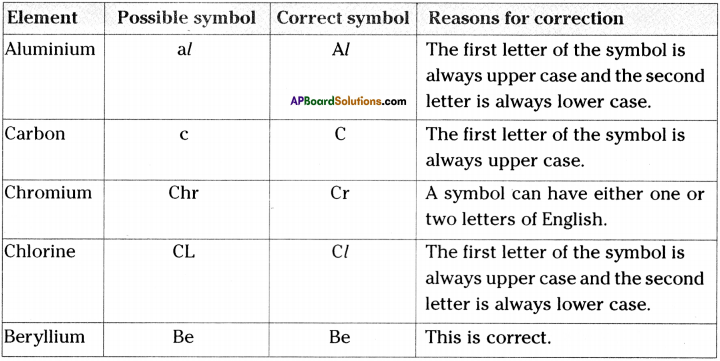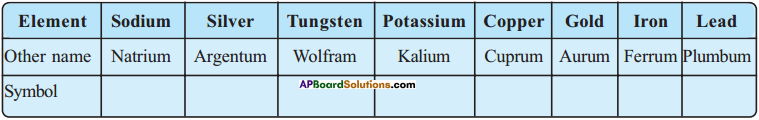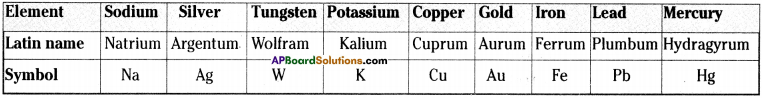AP State Syllabus AP Board 9th Class Physical Science Solutions Chapter 4 Atoms and Molecules Textbook Questions and Answers.

## AP State Syllabus 9th Class Physical Science Solutions 4th Lesson Atoms and Molecules

### 9th Class Physical Science 4th Lesson Atoms and Molecules Textbook Questions and Answers

Question 1.
Draw the diagram to show the experimental setup for the law of conservation of mass. (AS 5)
(OR)
Draw the experimental arrangement used in verifying law of conservation of mass. Write the law of conservation of mass.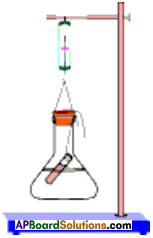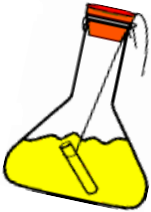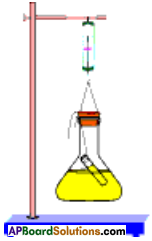Question 2.
Explain the process and precautions in verifying law of conservation of mass. (AS 5)
(OR)
Explain the procedure to prove in a chemical reaction the mass neither destroyed.Aim :
To verify law of conservation of mass.

Material required :
Sodium sulphate,Barium chloride, distilled water, conical flask, spring balance, small test tube, rubber cork, thread, retort stand.

Procedure:

1. Prepare a solution of sodium sulphate by dissolving approximately 2 gm of sodium sulphate in 100 ml distilled water in a 250 ml conical flask.
2. Prepare a Barium chloride solution by dissolving approximately 2 gm of potassium iodide in 100 ml water in another conical flask.
3. Take 100 ml solution of sodium sulphate in 250 ml conical flask.
4. Also take 4 ml solution of Barium chloride in test tube.
5. Hang the test tube in the flask carefully without mixing the solutions. Put a cork on the flask.
6. Weigh the flask with its contents carefully by spring balance.
7. Now tilt and swirl the flask, so that the two solutions mix.
8. Weigh the flask again by the spring balance.

Observations:

1. Weight of flask and contents before mixing = m1 g
2. Weight of flask and contents after mixing = m2 g

Conclusion :

1. We have observed that the two weights i.e., mj and m2 are equal.
2. This proves the law of conservation of mass.

Precautions:

1. Care should be taken while handling chemicals.
2. Glass apparatus may slip and break down. Hence make sure that they should not slip from your hands.
3. Contents of the conical, ffhsk should not mix before weighing first time.
4. Tie a thick thread to the conical flask, so that it will not slip while weighing.Question 3.
15.9g of copper sulphate and 10.6g of sodium carbonate react together to give 14.2g of sodium sulphate and 12.3 g of copper carbonate. Which law of chemical combination is obeyed? How? (AS 1, AS 2)
Reactants:
Mass of copper sulphate = 15.9 g ; Mass of sodium carbonate = 10.6 g
Total mass of reactants = 15.9 + 10.6 = 26.5 g

Products:
Mass of sodium sulphate = 14.2 g ; Mass of copper carbonate = 12.3 g
Total mass of products = 14.2 + 12.3 = 26.5 g
∴ Total mass of reactants is equal to total mass of products. This is the “Law of conservation of Mass”.

Question 4.
Carbon dioxide is added to 112 g of calcium oxide. The product formed is 200 g of calcium carbonate. Calculate the mass of carbon dioxide used. Which law of chemical combination will govern your answer? (AS 1, AS 2)

1. Let x g of carbon dioxide is added to 112 g of calcium oxide.
2. The product is 200 g of calcium carbonate.
3. According to law of conservation of mass, ,
Total mass of reactants = Total mass of products
x+ 112 = 200 g
x = 88 g
∴ 88 g of carbon dioxide is used.

Question 5.
0.24 g sample of compound of oxygen and boron was found by analysis to contain 0.144 g of oxygen and 0.096 g of boron. Calculate the percentage composition of the compound by weight. (AS 1)
Mass of compound of oxygen and boron = 0.24 g
On analysis,
Mass of oxygen in the compound = 1.44 g
Mass of boron in the compound = 0.096 g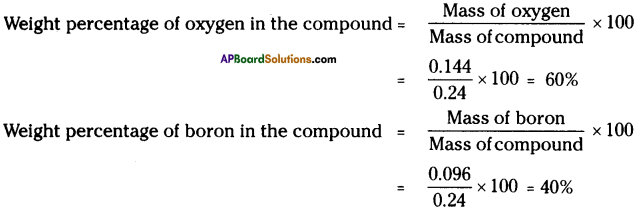Question 6.
In a class, a teacher asked students to write the molecular formula of oxygen. Shamita wrote the formula as 02 and Priyanka as O. Which one is correct? State the reason. (AS 1, AS 2)
Reason :

1. Oxygen is diatomic.
2. Two atoms of oxygen combine to form oxygen molecule.
3. Hence the formula of oxygen molecule will be ‘O2‘.Question 7.
Imagine what would happen if we do not have standard symbols for elements. (AS 2)
(OR)
Is it necessary to use symbols for elements? Write your opinion.

• Chemistry involves a lot of reactions.
• If we do not have symbols, we have to write their names to represent the reactions.
• This is very tedious work.
• To avoid this, we need standard symbols to elements, which are universally accepted.
• In advanced studies, balancing of equations, atoms present in a compound, etc. will not be understood without symbols.
• Simply chemistry will not be developed unless symbols, formulae, etc. are not known.

Question 8.
Mohith said “H2 differs from 2H.” Justify. (AS 1)
H2 is the hydrogen molecule in which two hydrogen atoms are combined to form one hydrogen molecule.

2H is the hydrogen atom. Here 2 hydrogen atoms are ready to participate in chemical reaction.

Question 9.
Lakshmi gives a statement “CO and Co both represent element”. Is it correct? State reason. (AS 1, AS 2)
Lakshmi’s statement is incorrect.

Reason :

1. CO stands for carbon monoxide, a compound, which consists carbon and oxygen atoms.
2. This can be identified with the help of both C and O are capital (upper case) letters.
3. Co stands for cobalt, an element.
4. This can be identified with the help of ‘C’ is capital (upper case) letter and ‘o’ small (lower case) letter.Question 10.
The formula of water molecule is H2O. What information do you get from this formula? (AS 1)

• Water is a combination of hydrogen and oxygen.
• Two hydrogen atoms and one oxygen atom combine to form one water molecule.
• Molecular weight of water molecule is 18. [Hydrogen 1, Oxygen 16. H2O ⇒ 2 × 1 + 16=18]
• 18 g of water molecule contains 6.022 × 1023 particles in it.
• Valency of hydrogen is 1 and oxygen is 2.

Question 11.
How would you write 2 molecules of Oxygen and 5 molecules of Nitrogen? (AS 1)
2 molecules of oxygen → 2O2
Reason :

1. Oxygen is diatomic element.
2. Two oxygen atoms combine to form one oxygen molecule.
3. The formula of oxygen molecule is O2.

5 molecules of nitrogen → 5N2
Reason :

1. Nitrogen is also diatomic element.
2. Two nitrogen atoms combine to form one nitrogen molecule.
3. Molecular formula of nitrogen is N2.

Question 12.
The formula of a metal oxide is MO. Then write the formula of its chloride. (AS 1)

• The valency of oxide is 2 i.e., O-2.
• The formula of a metal oxide is given as MO.
• Hence the valency of the given metal must be 2 i.e., M+2.
• Valency of chloride is 1 i.e., C.
• Therefore according to criss-cross method, the formula of given metal chloride will be MCl2.

Question 13.
Formula of calcium hydroxide is Ca(OH)2 and zinc phosphate is Zn3(PO4)2. Then write the formula to calcium phosphate. (AS 1)
(OR)
Formula of calcium hydroxide is Ca(OH)2 and zinc phosphate is Zn3(PO4)2. Then write the valencies of calcium and phosphate and then write the formula of calcium phosphate.

• Formula of calcium hydroxide is Ca(OH)2.
• From criss-cross method we know that the valency of calcium is 2 i.e., Ca+2 and hydroxide is 1 i.e., OH.
• Formula of zinc phosphate is Zn3(PO4)2.
• Valency of Zn is 2 i.e., Zn+2, and valency of phosphate is 3 i.e., PO4-3.
• Now the formula of calcium phosphate according to criss-cross method is Ca3(PO4)2.Question 14.
Find out the chemical names and formulae for the following common household substances. (AS 1)
a) Common salt
b) Baking soda
c) Washing soda
d) Vinegar

 Common household substance Chemical name Formula a) Common salt Sodium chloride NaCl b) Baking soda Sodium bicarbonate NaHCO3 c) Washing soda Sodium carbonate Na2CO3 d) Vinegar Impure dilute acetic acid CH3COOH

Question 15.
Calculate the mass of the following. (AS 1)
a) 0.5 mole of N2 gas
b) 0.5 mole of N atoms
c) 3.011 × 1023 number of N atoms
d) 6.022 × 1023 number of N2 molecules
a) 0.5 mole of N2 gas :
Mass of one mole of N2 gas = 28 g. (∵ Molecular wt. of N2 = 28)
Mass of 0.5 mole of N2 gas = 28 × 0.5 = 14 g

b) 0.5 mole of N atoms :
Mass of one mole of N atoms = 14 g (∵ Atomic wt. of N = 14)
Mass of 0.5 mole of N atoms = 14 × 0.5 = 7 g

c) 3.011 × 1023 number of N atoms :
Mass of 6.022 × 1023 number of N atoms = 14 g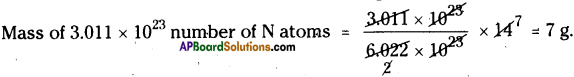d) 6.022 × 1023 number of N2 molecules :
Mass of 6.022 × 1023 number of N2 molecules = 28 g

Question 16.
Calculate the number of particles in each of the following. (AS 1)
a) 46 g of Na
b) 8 g of O2
c) 0.1 mole of hydrogen
a) 46 g of Na :
Atomic weight of Na = 23
Number of particles in 23 g of Na atom = 6.022 × 1023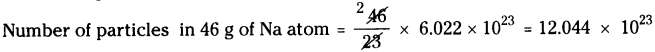b) 8 g of O2 :
Molecular weight of O2 is 32.
Number of particles in 32 g of O2 molecule = 6.022 × 1023c) 0.1 mole of hydrogen :
Atomic weight of hydrogen is 1.
Number of particles in 1 mole of hydrogen = 6.022 × 1023
Number of particles in 0.1 mole of hydrogen= $$\frac{0.1}{1}$$ × 6.022 × 1023 = 6.022 × 1022

Question 17.
Convert into moles. (AS 1)
a) 12 g of O2 gas
b) 20 g of water
c) 22 g of carbon dioxide
a) 12 g of O2 gas :
Molecular weight of O2 is 32.
∴ Number of moles of 32 g of O2 gas = 1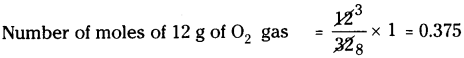b) 20 g of water :
Molecular weight of water (H2O) is 18.
Number of moles of 18 g of water = 1
Number of moles of 20 g of water = $$\frac{20}{18}$$ x 1 =1.11

c) 22 g of carbon dioxide :
Molecular weight of carbon dioxide (CO2) is 44.
∴ Number of moles of 44 g of CO2 = 1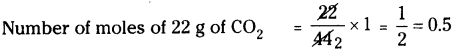Question 18.
Write the valencies of Fe in FeCl2 and FeCl3. (AS 1)

1. In FeCl2, the valency of Fe is 2.
2. In FeCl3, the valency of Fe is 3.

Question 19.
Calculate the molar mass of sulphuric acid (H2SO4) and glucose (C6H12O6). (AS 1)
a) Formula of sulphuric acid is H2SO4.
Molecular mass of H2SO4 = 2 × 1 + 1 × 32 + 4 × 16 = 2 + 32 + 64 = 98 u
∴ Molar mass of H2SO4 = 98 g

b) Formula of glucose is C6H12O6.
Molecular mass of C6H12O6 = (6 × 12) + (12 × 1) + (6 × 16) = 72 + 12 + 96 = 180 u
∴ Molar mass of C6H12O6 = 180 g.Question 20.
Which has more number of atoms – 100 g of sodium or 100 g of iron? Justify your answer. (Atomic mass of sodium = 23 u, atomic mass of iron = 56 u) (AS 1)
100g of sodium has more number of atoms than 100g of iron.

Justification :
1) Atomic mass of sodium = 23 u
23 g of sodium contains 6.022 × 1023 atoms.
100 g of sodium contains = $$\frac{100}{23}$$ × 6.022 × 1023 = 26.1826 × 1023 atoms of sodium.

2) Atomic mass of iron = 56 u
∴ 56 g of iron contains 6.022 × 1023 atoms.
100 g of iron contains = $$\frac{100}{56}$$ × 6.022 × 1023 = 10.7535 × 1023 atoms of iron.

Question 21.
Complete the following table. (AS 1)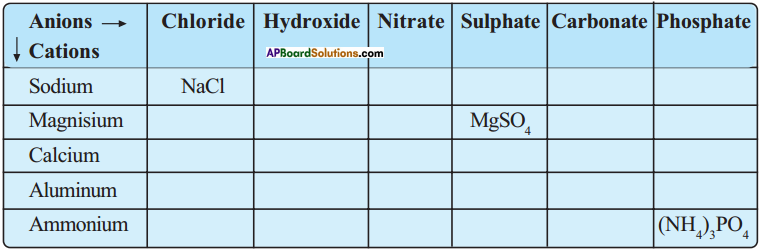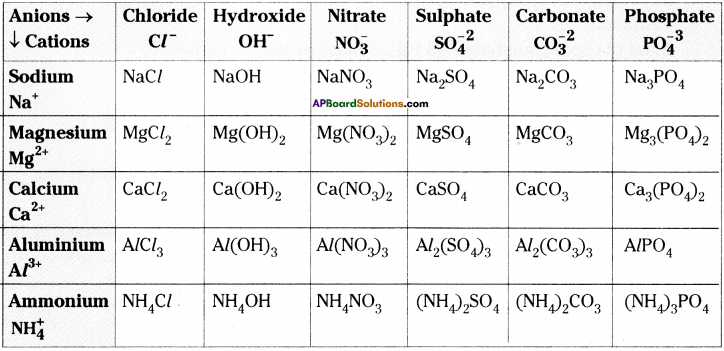Question 22.
Fill the following table. (AS 1)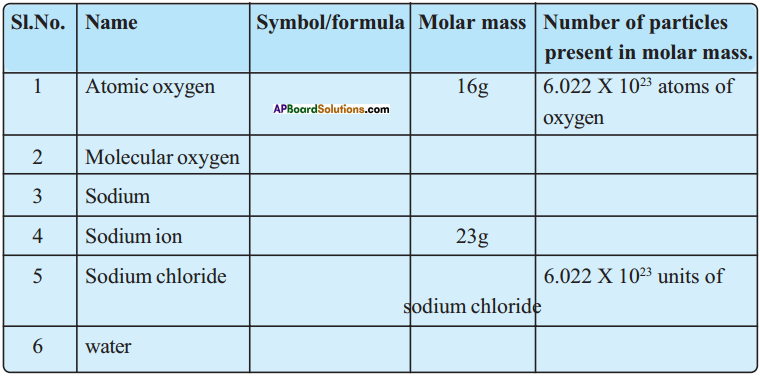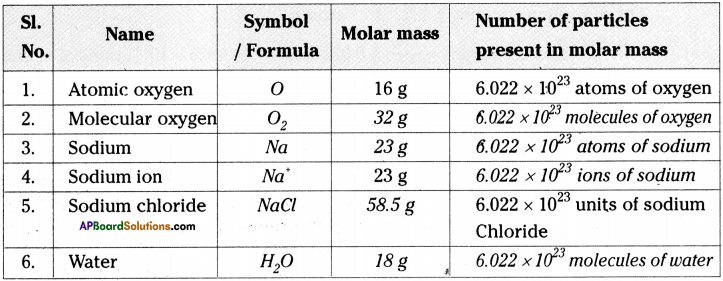Question 23.
Make placards with symbols and valencies of the atoms of the elements separately. Each student should hold two placards, one with the symbol in the right hand and the other with the valency in the left hand. Keeping the symbols in place, students should criss-cross their valencies to form the formula of a compound.
Student’s activity.

Question 24.
Take empty blister packs of medicines. Cut them into pieces having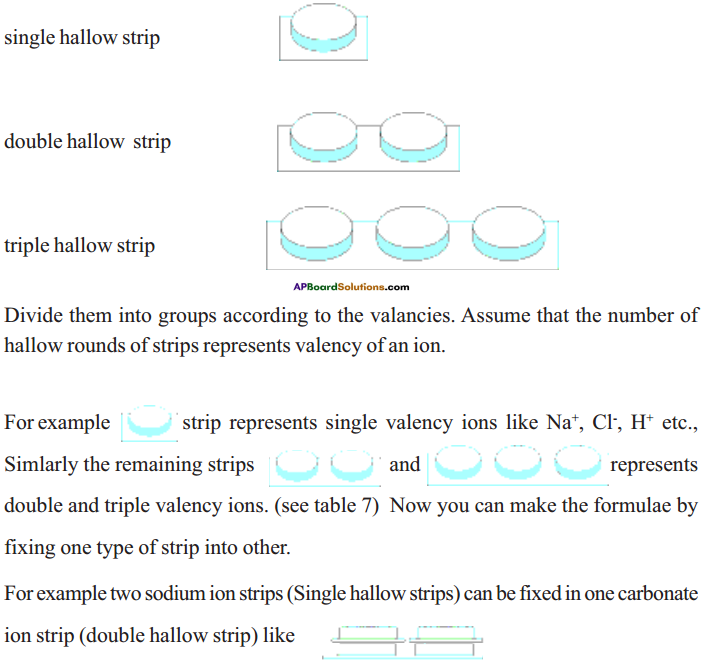Hence the formula of sodium carbonate will be Na2 CO3
Hence the forrnu A. Student’s activity.

### 9th Class Physical Science 4th Lesson Atoms and Molecules InText Questions and Answers

9th Class Physical Science Textbook Page No. 56

Question 1.
Does the weight of iron rod increase or decrease, on rusting?
The weight of iron rod decreases on rusting.

Question 2.
Where does the matter charcoal go?
The charcoal, on burning, gives off CO2 which is mixed in atmosphere. The residue is remained as ash.Question 3.
Wet clothes dry after some time – where does the water go?
Water evaporates and mixed in the atmosphere.

Question 4.
What happens to magnesium on burning it in air?
Magnesium on burning in air gives a bright light and ash is remained. The ash is magnesium oxide.

Question 5.
What happens to sulphur on burning it in air?
Sulphur on burning, changes its state and colour.

9th Class Physical Science Textbook Page No. 57

Question 6.
Did you observe any precipitate in the reaction?
In the flask, a reaction takes place between lead nitrate and potassium iodide.Question 7.
Do you think that a chemical reaction has taken place in the flask? Give reason.
Yes, the contents in the flask are changed as lead iodide and potassium nitrate.

9th Class Physical Science Textbook Page No. 58

Question 8.
Do the weights of the flask and its contents change during the activity?
The weights of the flask and its contents do not change before and after reaction.

Question 9.
Mass was neither created nor destroyed.Question 10.
What do you observe from table – 1?
The components of a compound are mixed at same proportions in any sample.

9th Class Physical Science Textbook Page No. 59

Question 11.
What difference do you observe in percentage of copper, carbon, and oxygen in two samples?
The percentage of copper, carbon and oxygen are same in two samples, i.e., they are mixed at same proportions.

9th Class Physical Science Textbook Page No. 60

Question 12.
Are elements also made of atoms?
When the particles of a substance contain only one type of atoms, that substance is called an element. In elements the smallest particle that exist may be atoms or molecules.

9th Class Physical Science Textbook Page No. 62

Question 13.
How do we write the symbols for calcium, chlorine, chromium?
We have only 26 alphabets in English, but there are over 100 known elements. We cannot write the same symbol for carbon, calcium, chromium, etc.

9th Class Physical Science Textbook Page No. 63

Question 14.
Would you be able to recognise the elements of the table – 2, have symbols of this category?
Yes. They are iron, gold, sodium, and potassium.

9th Class Physical Science Textbook Page No. 64

Question 15.
Observe the atomicity and fill the following table.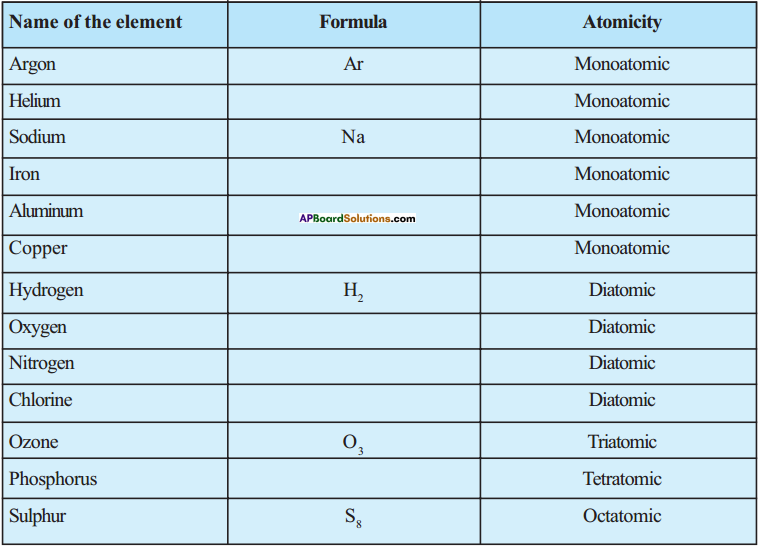Name of the element Formula Atomicity Argon Ar Monoatomic Helium He Monoatomic Sodium Na Monoatomic Iron Fe Monoatomic Aluminium Al Monoatomic Copper Cu Monoatomic Hydrogen H2 Diatomic Oxygen O2 Diatomic Nitrogen N2 Diatomic Chlorine Cl2 Diatomic Ozone O3 Triatomic Phosphorus P4 Tetratomic Sulphur S8 Octatomic

Question 16.
What is valency?
Every element reacts with other element according to its combining capacity, which we call as its valency.

9th Class Physical Science Textbook Page No. 66

Question 17.
Can you write the formula of carbon dioxide and carbon monoxide? Try to write formula for them as we have done in case of water molecule.
Carbon dioxide :
The elements present are carbon and oxygen. One atom of carbon and one atom of oxygen are present in a molecule of carbon monoxide. Hence the formula of carbon monoxide is CO.Carbon dioxide :
The elements present are carbon and oxygen. One atom of carbon and 2 atoms of oxygen are present in a molecule of carbon dioxide. Hence the formula of carbon dioxide is CO2.

9th Class Physical Science Textbook Page No. 69

Question 18.
How many molecules are there in 18 grams of water?
6.022 × 1023 molecules are there in 18 grams of water.

Question 19.
How many atoms are there in 12 grams of carbon?
6.022 × 1023 atoms are there in 12 grams of carbon.

9th Class Physical Science Textbook Page No. 58

Question 20.
Do you get the same result if the conical flask is not closed?

1. No, we cannot get the same result.
2. When the conical flask is not closed, some gases will leave out the flask during chemical reaction.Question 21.
Recall the burning of the magnesium ribbon in air. Do you think mass is conserved during this reaction?

1. Yes, but we cannot observe the conservation of mass.
2. When the experiment is conducted in a closed container where there is no scope for oxygen to escape, we can observe the conservation of mass. But in this condition this experiment is not possible.

9th Class Physical Science Textbook Page No. 59

Question 22.
100 g of mercuric oxide decompose to give 92.6 g of mercury and 7.4 g of oxygen. Let us assume that 10 g of oxygen reacts completely with 125 g of mercury to give mercuric oxide. Do these values agree with the law of constant proportions?
Proportion of oxygen = 7.4 : 10
Proportion of mercury = 92.6 : 125
$$\Rightarrow \frac{7.4}{10}=\frac{92.6}{125} \Rightarrow 0.74=0.74$$
∴ They follow law of constant proportions.

Question 23.
Discuss with your friends if the carbon dioxide that you breathe out and the carbon dioxide they breathe out are identical. Is the composition of the carbon dioxide of different sources same? (Page – 73)
Same.
This can be justified with the help of law of constant proportions.

9th Class Physical Science Textbook Page No. 60

Question 24.
Which postulate of Dalton’s theory is the result of the law of conservation of mass?
First postulate of Dalton’s theory i.e. “Matter consists of indivisible particles called atoms”, is the result of law of conservation of mass.Question 25.
Which postulate of Dalton’s theory can explain the law of constant proportions?
Third postulate of Dalton’s theory i.e. “Atoms of a given element have identical mass and chemical properties. Atoms of different elements have different masses and chemical properties”, is the result of law of constant proportions.

9th Class Physical Science 4th Lesson Atoms and Molecules Activities

Activity – 1

Question 1.
Some elements and their possible symbols are given. Correct them and give reasons for your corrections.